Minmin Chen、Alex Beutel等在《Top-K Off-Policy Correction for a REINFORCE Recommender System》中提出使用强化学习来提升youtube推荐。主要是从bias/variance的角度出发，具体方法如下：

# 摘要

• 1.将REINFORCE扩展到生产环境推荐系统上，动作空间有数百万；
• 2.使用off-policy correction来解决在从多种行为策略中收集的日志反馈的数据偏差（data biases）
• 3.提出了一种新的top-K off-policy correction来解释我们一次推荐多个items的策略推荐
• 4.展示了探索(exploration)的价值

# 1.介绍

• 2.Off-Policy候选生成：我们使用off-policy correction来从日志反馈中学习，这些日志从之前的model policies的一个ensemble中收集而来。我们会结合一个已经学到的关于行为策略(behavior policies)的神经网络模型来纠正数据偏差。
• 3.Top-K Off-policy Correction：我们提供了一个新的top-K off-policy correction来说明：我们的推荐一次输出多个items。
• 4.真实环境的提升：我们展示了在真实环境中（在RL文献中很少有这种情况），这些方法对于提升用户长期满意度的价值。

# 3.增强推荐• S：用于描述用户状态(user states)的一个连续状态空间（state space）
• A：一个离散的动作空间(action space)，它包含了推荐可提供的items
• $P: S \times A \times S \rightarrow R$：是一个状态转移概率
• $R: S \times A \rightarrow R$：回报函数(reward function)，其中$r(s,a)$是立即回报(immediate reward)，它通过在用户状态（user state）s上执行动作a得到
• $\rho_0$：初始state分布
• $\gamma$：对于future rewards的discount factor

$max_{\pi} E_{\tau \sim \pi} [R(\tau)], where \ R(\tau) = \sum\limits_{t=0}^{|\tau|} r(s_t, a_t)$$E_{\tau \sim \pi_\theta} [R(\tau) \nabla_\theta log \pi_{\theta} (\tau)]$

…(1)

$\sum_{\tau \sim \pi_{\theta}} R(\tau) \nabla_{\theta} log \pi_{\theta}(\tau) \approx \sum_{\tau \sim \pi_{\theta}} [ \sum_{t=0}^{|\tau|} R_t \nabla_{\theta} log \pi_{\theta} (a_t | s_t)]$

…(2)

# 4.off-policy collrection

$\sum_{\tau \sim \beta} \frac{\pi_{\theta}(\tau)}{\beta(\tau)} [\sum_{t=0}^{|\tau|} R_t \nabla_{\theta} log(\pi_{\theta} (a_t | s_t))]$

$\frac{\pi_{\theta}(\tau)}{\beta(\tau)} = \frac{\rho(s_0) \prod_{t=0}^{|\tau|} P(s_{t+1}|s_t, a_t) \pi(a_t|s_t)}{\rho(s_0) \prod_{t=0}^{|\tau|} P(s_{t+1}|s_t, a_t) \beta(a_t | s_t)} = \prod_{t=0}^{|\tau|} \frac{\pi(a_t | s_t)}{\beta(a_t | s_t)}$

$\prod_{t'=0}^{|\tau|} \frac{\pi(a_{t'} | s_{t'})}{\beta(a_{t'} | s_{t'})} \approx \prod_{t'=0}^{t} \frac{\pi(a_{t'} | s_{t'})}{\beta(a_{t'}|s_{t'})} = \frac{P_{\pi_{\theta}}(s_t)}{P_{\beta}(s_t)} \frac{\pi(a_t | s_t)}{\beta(a_t|s_t)} \approx \frac{\pi(a_t|s_t)}{\beta(a_t | s_t)}$

$\sum_{\tau \sim \beta} [\sum_{t=0}^{|\tau|} \frac{\pi_{\theta} (a_t |s_t)}{\beta(a_t | s_t)} R_t \nabla_{\theta} log \pi_{\theta} (a_t | s_t)]$

…(3)

Achiam证明了：该一阶近似对于学到的policy上的总回报的影响，会通过$O(E_{s \sim d^{\beta}} [D_{TV}(\pi \mid \beta)[s]])$来限定幅值，其中$D_{TV}$是在$\pi(\cdot \mid s)$和$\beta(\cdot \mid s)$间的总方差，$d^{\beta}$是在$\beta$下的discounted future state分布。该estimator会权衡精确的off-policy correction的方差，并仍能为一个non-corrected policy gradient收集大的偏差，这更适合on-policy learning。

## 4.1 对policy $\pi_{\theta}$进行参数化

• user state （$s_t \in R^n$）: 我们对每个时间t上的user state建模，这可以同时捕获用户兴趣的演进，它使用n维向量$s_t \in R^n$来表示。
• action （$u_{a_t} \in R^m$）: 沿着该轨迹（trajectory）每个时间t上的action会使用一个m维向量$u_{a_t} \in R^m$进行嵌入。

$s_{t+1} = f(s_t, u_{a_t})$

$s_{t+1} = z_t \odot tanh(s_t) + i_t \odot tanh(W_a u_{a_t}) \\ z_t = \sigma(U_z s_t + W_z u_{a_t} + b_z) \\ i_t = \sigma(U_i s_t + W_i u_{a_t} + b_i)$

…(4)

• $z_t \in R^n$是update gate
• $i_t \in R^n$是input gate

$\pi_{\theta}(a | s) = \frac{exp(s^{\top} v_a / T)}{\sum\limits_{a' \in A} exp(s^{\top} v_{a'} / T)}$

…(5)

• $v_a \in R^n$是每个action a在action space A中的另一个embedding（注：前面的$u_{a_t} \in R^m$是m维，而$v_a$是与$s_t$相同维度）
• T是时序(通常设置为1)。T值越大会在action space上产生一个更平滑的policy。

• 两个action embeddings：$U \in R^{m \times \mid A \mid}$和$V \in R^{n \times \mid A \mid}$，
• 在RNN cell中的权重矩阵：$U_z, U_i \in R^{n \times n}, W_u, W_i, W_a \in R^{n \times m}$
• biases：$b_u, b_i \in R^n$## 4.2 估计behavior policy $\beta$

• (1) 在我们的系统中有许多agents，许多是不可控的
• (2) 一些agents具有一个deterministic policy，将$\beta$设置成0或1并不是使用这些日志反馈(logged feedback)的最有效方式

• (1) main policy $\pi_{\theta}$会使用一个weighted softmax来考虑长期回报(long term reward)进行有效训练；而behavior policy head $\beta_{\theta'}$只会使用state-action pairs进行训练（言下之意，不考虑reward?）
• (2) main policy head $\pi_\theta$只使用在该trajectory上具有非零回报（non-zero reward）的items进行训练(注3)；而behavior policy $\beta_{\theta'}$使用在该轨迹上的所有items进行训练，从而避免引入在$\beta$估计时的bias

## 4.3 Top-K off-policy Correction

$max_{\theta} E_{\tau \sim \prod_{\theta}} [ \sum\limits_t r(s_t, A_t)]$

$\prod_{\theta}(A' | s) = \prod\limits_{a \in A'} \pi_{\theta} (a | s)$

$\sum_{\tau \sim \pi_\theta} [ \sum_{t=0}^{|\tau|} R_t \nabla_{\theta} log \alpha_{\theta} (a_t | s_t)]$

• $\alpha_{\theta} (a \mid s) = 1 - (1- \pi_{\theta}(a \mid s))^K$：表示的是一个item a出现在最终的无重复集合A中的概率。这里，$K = \mid A'\mid >\mid A\mid = k$。（注：作为有放回(replacement)和去重（de-duplicate）抽样的结果，最终集合A的size是可变的）

$\sum_{\tau \sim \beta} [ \sum_{t=0}^{|\tau|} \frac{\alpha_{\theta} (a_t |s_t)}{\beta(a_t|s_t)} R_t \nabla_{\theta} log \alpha_{\theta} (a_t | s_t)] \\ = \sum_{\tau \sim \beta} [\sum_{t=0}^{|\tau|} \frac{\pi_{\theta}(a_t|s_t)}{\beta(a_t|s_t)} \frac{\partial \alpha(a_t|s_t)}{\partial \pi(a_t|s_t)} R_t \nabla_{\theta} log \pi_{\theta} (a_t | s_t)]$

…(6)

$\lambda_K(s_t, a_t) = \frac{\partial \alpha(a_t | s_t)}{\partial \pi(a_t | s_t)} = K(1-\pi_{\theta} (a_t | s_t))^{K-1}$

…(7)

• 随着$\pi_{\theta}(a\mid s) \rightarrow 0, \lambda_K(s,a) \rightarrow K$。对比起标准的off-policy correction，top-K off-policy correction会通过一个K因子来增加policy update；
• 随着$\pi_{\theta}(a \mid s) \rightarrow 1, \lambda_K(s,a) \rightarrow 0$。该乘子会使policy update归0
• 随着K的增加，以及$\pi_{\theta}(a \mid s)$会达到一个合理的范围, 该乘子会更快地将graident减小于0

## 4.4 Variance Reduction技术

Weight Capping。

$\omega_c(s,a) = min(\frac{\pi(a|s)}{\beta(a|s)}, c)$

…(8)

c的值越小，会减小在梯度估计时的方差，但会引入更大的bias。

NIS(归一化重要性抽样：Normalized Importance Sampling)

$\bar{\omega}(s, a) = \frac{w(s,a)}{\sum\limits_{(s',a') \sim \beta} w(s', a')}$

TRPO(Trusted Region Policy Optimization). TRPO会阻止new policy $\pi$背离behavior policy，它通过增加一个正则项来惩罚这两个policies的KL散度。它会达到与weight capping相似的效果。

# 6.实验结果

## 6.1 仿真

### 6.1.1 off-policy correction

$\pi^* (a_i) = I(i=10)$

$\pi(a_i) = \frac{e^{\theta_i}}{\sum_j e^{\theta_j}}$

$\pi(a_i) = \frac{r(a_i)\beta(a_i)}{\sum_j r(a_j) \beta(a_j)}$actions = [1,2,3,4,5,6,7,8,9,10]
b = lambda x: (11-x)/55.0
beta = [b(i) for i in actions]
rxb = [(i+1)*j for i, j in enumerate(beta)]
total = sum(rxb)
pi = [i/total for i in rxb]



### 6.1.2 Top-K-policy correction

$\theta_j = \theta_j + \eta \frac{\pi_{\theta}(a_j)}{\beta(a_j)} r(a_i) [ I(j=i) - \pi_{\theta}(a_j)] , \forall j = 1, \cdots, 10$

$\theta_j = \theta_j + \eta \lambda_K(a_i) \frac{\pi_\theta(a_j)}{\beta(a_j)} r(a_i) [I(j=i) - \pi_{\theta}(a_j)], \forall j=1, \cdots, 10$## 6.2 真实环境

### 6.2.2 Off-policy Correction

【注6】：实际中，我们使用一个相当小比例的用户做为test polulation；作为结果，我们记录的feedback则几乎通过control model获取actions数目

Capping

# PPO(openai)介绍

openai在《Proximal Policy Optimization Algorithms》提出了PPO。我们来看下：

# 2.背景: Policy Optimization

$\hat{g} = \hat{E}_t [ \nabla_{\theta} log \pi_{\theta} (a_t | s_t) \hat{A}_t ]$

…(1)

• $\pi_{\theta}$是一个stochastic policy
• $\hat{A}$是一个在timestep t时advatage function的estimator
• 期望$\hat{E}_t[\cdots]$表示在一个会在sampling和optimization间做交替的算法中，一个有限batch的样本上的经验平均(empirical average)。

$L^{PG}(\theta) = \hat{E}_t [log \pi_{\theta} (a_t | s_t) \hat{A}_t]$

…(2)

## 2.2 Trust Region方法

$\underset{\theta}{maximize} \ \hat{E}_t [\frac{\pi_{\theta}(a_t|s_t)}{\pi_{\theta_{old}}(a_t|s_t)} \hat{A}_t ]$

$\hat{E}_t [KL[\pi_{\theta_{old}}(\cdot | s_t) , \pi_{\theta}(\cdot|s_t)]] \leq \delta$

…(4)

TRPO的理论证明建议我们使用一个正则项(penalty)来替代constraint，比如，对一些系数$\beta$求解以下没有constraint的最优化问题：

$\underset{\theta}{maximize} \ \hat{E}_t [\frac{\pi_{\theta}(a_t|s_t)}{\pi_{\theta_{old}}(a_t | s_t)} \hat{A}_t - \beta KL[\pi_{old}(\cdot|s_t), \pi_{\theta}(\cdot|s_t)]]$

…(5)

# 3.对Surrogate Objective进行裁减（Clip）

$L^{CPI}(\theta) = \hat{E}_t [\frac{\pi_{\theta}(a_t |s_t)}{\pi_{\theta_{old}}(a_t | s_t)} \hat{A}_t] = \hat{E}[r_t(\theta) \hat{A}_t]$

…(6)

$L^{CLIP}(\theta) = \hat{E}_t [min(r_t(\theta) \hat{A}_t, clip(r_t(\theta), 1-\epsilon, 1+\epsilon) \hat{A}_t)]$

…(7)• 1.使用一些minibatch SGD的epochs，来优化KL-penalized objective：$L^{KLPEN}(\theta) = \hat{E}_t [\frac{\pi_{\theta}(a_t \mid s_t)}{\pi_{\theta_{old}}(a_t \mid s_t)} \hat{A}_t - \beta KL [\pi_{\theta_{old}} (\cdot \mid s_t), \pi_{\theta}(\cdot \mid s_t)] ]$
• 计算 $d = \hat{E}_t[KL[\pi_{\theta}(\cdot \mid s_t), \pi_{\theta}(\cdot \mid s_t)]]$
• if $d < d_{targ} / 1.5, \beta \leftarrow \beta / 2$
• if $d > d_{targ} \times 1.5, \beta \leftarrow \beta \times 2$

# 5.算法

$L_t^{CLIP+VF+S}(\theta) = \hat{E}_t [L_t^{CLIP}(\theta) - c_1 L_t^{VF}(\theta) + c_2 S[\pi_{\theta}](s_t)]$

….(9)

• $c_1, c_2$是系数
• S表示一个entropy bonus
• $L_t^{VF}$是一个squared-error loss：$(V_{\theta}(s_t) - V_t^{targ})^2$

$\hat{A}_t = -V(s_t) + r_t + \gamma r_{t+1} + \cdots + \gamma^{T-t+1} r_{T-1} + \gamma^{T-t} V(s_T)$

…(10)

$\hat{A}_t = \delta_t + (\gamma \lambda) \delta_{t+1} + \cdots + \cdots + (\gamma \lambda)^{T-t+1} \delta_{T-1}, \\ where \ \ \delta_t = r_t + \gamma V(s_{t+1}) - V(s_t)$

….(11)(12)# PPO(deepmind版)介绍

deepmind在《Emergence of Locomotion Behaviours in Rich Environments》提出了PPO。我们来看下：

# 2.使用Distributed PPO进行大规模增强学习

Policy gradient算法为连续控制（continuous control）提供了一个吸引人的范式。他们通过根据stochastic policy $\pi_{\theta}(a \mid s)$的参数$\theta$直接最大化rewards的期望和：

$J(\theta) = E_{\rho_{\theta}(\tau)} [\sum_t \gamma^{t-1} r(s_t, a_t)]$

$\rho_{\theta}(\tau) = p(s_0) \pi(a_0 | s_0) p(s_1 | s_0, a_0) ...$

$\theta$对应的目标函数的梯度是：

$\nabla_{\theta} J = E_{\theta} [\sum_t \nabla_{\theta} log \pi_{\theta}(a_t | s_t)(R_t - b_t)]$

• $R_t = \sum_{t'=t} \gamma^{t'-t} r(s_{t'}, a_{t'})$
• $b_t$是一个baseline（它不依赖于$a_t$或者future states和actions）。该baseline通常会选择$b_t = V^{\theta} (s_t) = E_{\theta} [R_t \mid s_t]$。

Policy gradient的estimates可以有很高的variance，算法对于超参数的设置很敏感。有许多方法可以使得policy gradient算法更健壮。一种有效的measure是：采用一个trust region constraint，可以限制policy update的任意更新量(amount)。采用该思想的一种流行算法是：TRPO（trust region policy optimization）。在每个迭代中，给定当前参数$\theta_{old}$，TRPO会收集一个（相对较大）的batch数据，并优化surrogate loss：

$J_{TRPO}(\theta) = [ \sum_t \gamma^{t-1} \frac{\pi_{\theta}(a_t | s_t)}{\pi_{\theta_{old}}(a_t | s_t)} A^{\theta_{old}}(a_t, s_t)]$

$KL[\pi_{\theta_{old}} | \pi_{\theta}] < \delta$

$A^{\theta}$是advantage function，可以通过$A^{\theta}(s_t, a_t) = E_{\theta}[R_t \mid s_t, a_t] - V^{\theta}(s_t)$得到。

PPO算法可以被看成是一个依赖于一阶梯度的TRPO的近似版本，它可以更方便地使用RNNs，并可以用在一个大规模分布式setting中。trust region constraint通过一个正则项来实现。使用的正则项系数依赖于该是否先前违反了constraint。算法1展示了PPO算法的伪代码。John Schulman的公开提供的PPO实现对于核心算法增加了一些修改。它们包含了对inputs、rewards、以及在loss中的一个额外项（它会惩罚对于较大违反trust region constraint的情况）的normalization。我们采用在分布式setting中相似的augmentations，但发现在跨多个workers间对多个统计进行sharing和synchronization需要一些注意。我们的分布式实现(DPPO)采用tensorflow，参数在一个parameter server中，workers会在每个gradient step后同步它们的参数。伪码和详情在附件中提供。

## A.DPPO

A.1 算法详情normalization

• 1.我们会通过减mean并除以标准差，将observations（或者 states $s_t$）进行归一化。。。
• 2.我们会通过一个正在运行的关于标准差的estimate对reward进行缩放（scale），在整个实验过程中进行聚合

# 1.介绍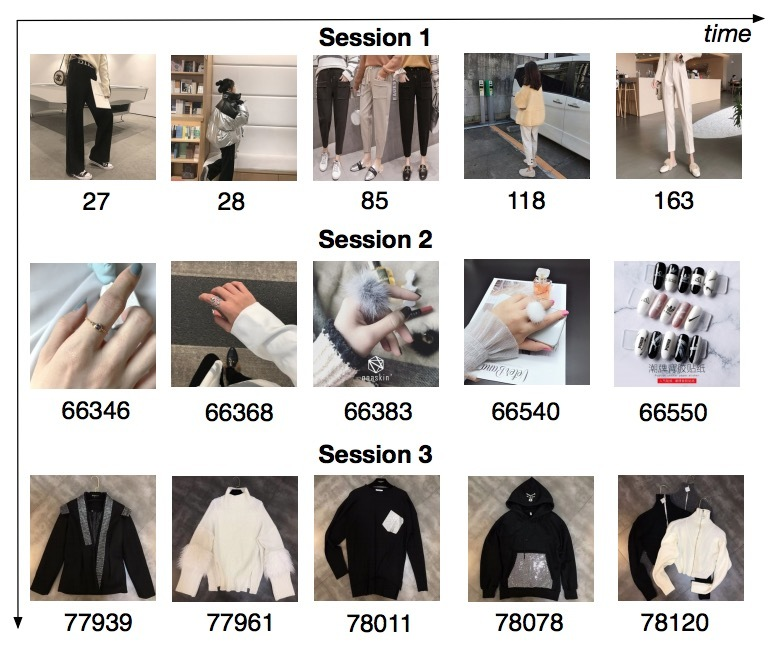• 首先，将用户序列行为划分成sessions
• 接着，使用self-attention network以及bias encoding来建模每个session。Self-attention可以捕获session行为(s)的内在交互/相关，接着抽取每个session的用户兴趣(s)。这些不同的session interests可能相互间相关，接着遵循一个序列模式。
• 最后，我们使用Bi-LSTM来捕获交互、以及用户多个历史session interests的演进。由于不同session interests对于target item具有不同的影响，最终我们设计了local activation unit根据target item来聚合他们，形成该行为序列的最终表示。

• 我们强调用户行为在每个session中高度同质，不同sessions差异很大。
• 设计了一个self-attention network以及bias encoding来获得每个session的精准兴趣表示。接着我们使用Bi-LSTM来捕获历史sessions间的顺序关系(sequential relationship)。最后，考虑到不同session interest在target item上的影响，我们使用local activation unit来聚合。
• 两组比较实验。表明DSIN效果更好。

# 2.相关工作

## 2.2 Session-based推荐

session的概率常被序列化推荐提及，但很少出现在CTR预测任务中。Session-based推荐受益于用户兴趣在sessions上动态演化的启发。GFF使用关于items的sum pooling来表示一个session。每个item具有两种表示，一个表示自身，另一个表示session的上下文(context)。最近，RNN-based方法被应用于session-based推荐中来捕获在一个session中的顺序关系。基于此，Li 2017提出了一个新的NARM（attentive neural networks framework）来建模用户的序列化行为，并能捕获用户在当前session中的主要目的。Quadrana 2017提出的Hierarchical RNN依赖于RNNs的latent hidden states跨用户历史sessions的演化。另外，Liu 2018 的RNNs使用self-attention based模型来有效捕获一个session的long-term和short-term兴趣。Tang 2018使用CNN、Chen 2018使用user memory network来增强序列模型的表现力。

# 3.DSIN

## 3.1 BaseModel

• feature representation,
• embedding,
• MLP以及loss function。

CTR预测任务中统计了大量信息特征。总共使用了三大组：User profile、item profile、User Behavior。每组包含了一些稀疏特征：

• User Profile包含了gender、city等；
• Item Profile包含了：seller id、brand id等；
• User Behavior包含了用户最近点击的item ids等

Embedding

MLP

Loss Function

## 3.2 模型总览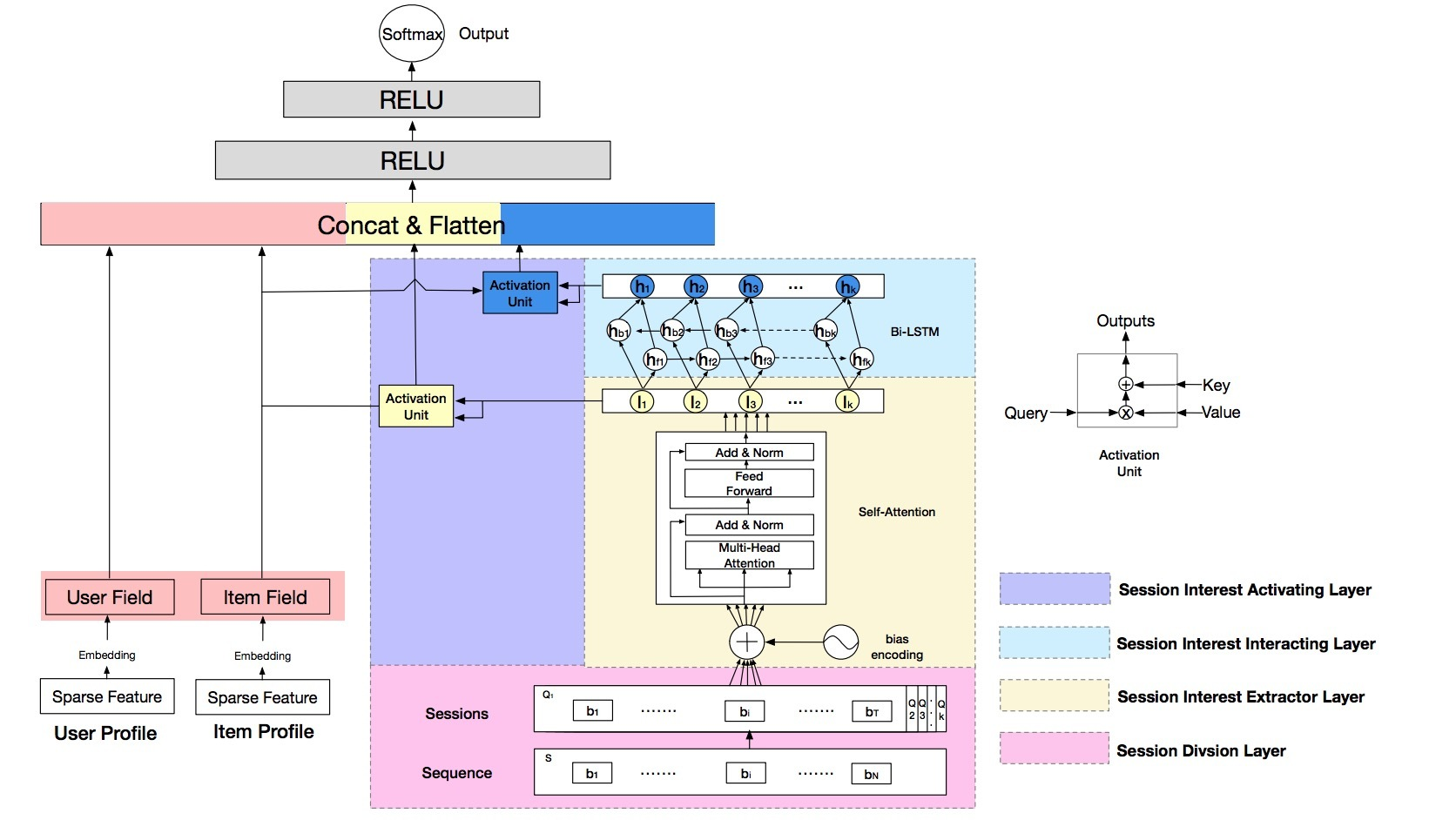• 1) session division layer，会将用户行为序列划分为sessions
• 2) session interest extractor layer：会抽取用户的session interests
• 3) session interest interacting layer：会捕获session interests间的顺序关系
• 4) session interest activating layer：会对与target item有关的session interests使用local activation unit

### 1.Session Division Layer

$Q_k = [b_1; \cdots; b_i; \cdots; b_T] \in R^{T \times d_{model}}$

• T是我们在该session中的行为数
• $b_i$是在该session中的用户第i个行为

### 2.Session Interest Extractor Layer

2-1 Bias Encoding

$BE \in R^{K \times T \times d_{model}}$

$BE_{(k,t,c)} = w_k^K + w_t^T + w_c^C$

…(2)

• $w^K \in R^K$：是session的bias vector
• k：是sessions的索引
• $w^T \in R^T$：是在session中position的bias vector
• t：是在sessions中行为的索引
• $w^C \in R^{d_{model}}$：是在behavior embedding中unit position的bias vector
• c：是在behavior embedding中unit的index

$Q = Q + BE$

…(3)

$Q_k = [Q_{k1}; \cdots; Q_{kh}; \cdots; Q_{kH}]$

• $Q_{kh} \in R^{T \times d_h}$：是$Q_k$的第h个head
• $d_h = \frac{1}{h} d_{model}$

$head_h$的输出如下计算：

$head_h = Attention(Q_{kh} W^Q, Q_{kh} W^K, Q_{kh} W^V) \\ =softmax(\frac{Q_{kh} W^Q W^{K^T} Q_{kh}^T}{\sqrt{d_{model}}}) Q_{kh} W^V$

…(4)

$I_k^Q = FFN(Concat(head_1, \cdots, head_H) W^O)$

…(5)

$I_k = Avg(I_k^Q)$

…(6)

### 3.Session Interest Interacting Layer

$i_t = \sigma(W_{xi} I_t + W_{hi} h_{t-1} + W_{ci} c_{t-1} + b_i) \\ f_t = \sigma(W_{xf} I_t + W_{hf} h_{t-1} + W_{cf} c_{t-1} + b_f) \\ c_t = f_t c_{t-1} + i_t tanh(W_{xc}I_t + W_{hc} h_{t-1} + b_c) \\ o_t = \sigma(W_{xo} I_t + W_{ho} h_{t-1} + W_{co}c_t + b_o) \\ h_t = o_t tanh(c_h)$

…(7)

$H_t = \overrightarrow {h_{ft}} \oplus \overleftarrow {h_{bt}}$

…(8)

### 4.Session Interest Activating Layer

$a_k^I = \frac{exp(I_k W^I X^I)}{\sum\limits_k^K exp(I_k W^I X^I)} \\ U^I = \sum\limits_k^K a_k^I I_k$

…(9)

$a_k^H = \frac{exp(H_k W^H X^I)}{\sum\limits_k^K exp(H_k W^H X^I)} \\ U^H = \sum\limits_k^K a_k^H H_k$

…(10)

# MIND召回介绍

#

tmall在《Multi-Interest Network with Dynamic Routing for Recommendation at Tmall》开放了它们的召回算法。在matching stage上，提出了Multi-Interest Network with Dynamic routing (MIND)来处理用户的多样化兴趣。特别的，还基于capsule routing机制设计了一个multi-interest extractor layer，用于聚类历史行为和抽取多样化兴趣。另外，我们还开发了一种称为”label-aware attention”的技术来帮助学习具有多个向量的用户表示 。目前的效果要好于state-of-the-art的其它推荐方法。并在天猫APP的移动端主页上部署，会处理主要的在线流量。

# 1.介绍

tmall APP的主页如图1(左）所示，它占据着tmall APP的半数流量，并会部署RS来展示个性化的商品来满足客户个性化需求。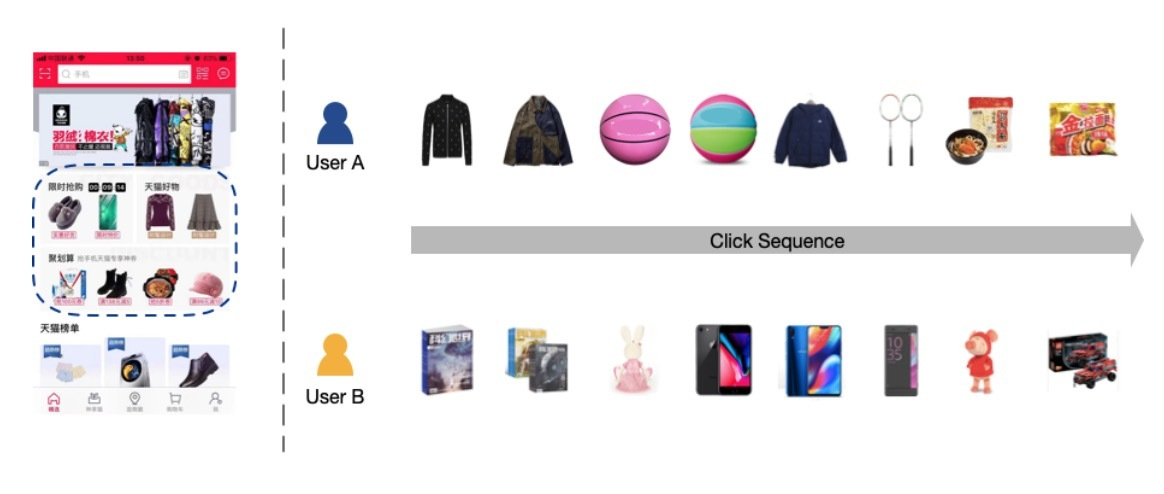• 1.为了捕获用户行为的多样化兴趣，我们设计了multi-interest extractor layer，它可以利用dyniamic routing来自适应地将用户历史行为聚合成用户表示向量。
• 2.通过使用由multi-interest extractor layer和一个新提出的label-aware attention layer生成的用户表示向量(vectors)，我们构建一个DNN来做个性化推荐。对比已存在的方法，MIND在多个公开数据集上和天猫上的效果要好一些。
• 3.为了在tmall上部署MIND，我们构建了一个系统来实现整个pipline，包括：data collecting、model training和online serving。部署的系统可以极大提升Tmall APP的ctr。

# 2.相关工作

User Representation。在推荐系统中，将users表示为vectors很常见。传统的方法会将用户偏好组合成由感兴趣的items[4,12,22]、keywords[5,8]和topics的vectors。随着分布式表示学习的出现，user embeddings可以通过NN获得。使用RNN-GRU来从时序阅读文档中学习user embeddings。从word embedding vectors中学习user embedding vectors，并将它们应用于推荐学术微博上。提出了一种新的CNN-based模型来显式学习和利用user embeddings。

Capsule Network。“胶囊（Capsule）”的概念，对一小部分neurons组合输出一整个vector，首次由2011年Hinton提出。用于替代BP，dynamic routing被用于学习capsules间连接的权重，通过利用EM算法来克服多种缺陷并达到较好的accuracy。它与CNN有两个主要不同之处，使得capsule networks可以编码在part和whole间的关系，这在CV和NLP中是很先进的。SegCaps证明，capsules可以成功建模目标的局部关系（spatial），比传统CNNs要好。研究了文本分类的capsule网络，并提出了3种策略来增强效果。

# 3.方法

## 3.1 问题公式化

• $I_u$表示与用户u交互的items集合（也称为：用户行为(user behavior)）
• $P_u$是用户u的基础profiles（比如：gender和age）
• $F_i$是target item的特征（比如：item id和category id）

MIND的核心任务是，学习一个函数，来将原始特征（raw features）映射到用户表示上，它可以公式化为：

$V_u = f_{user}(I_u, P_u)$

…(1)

$\vec{e}_i = f_{item}(F_i)$

…(2)

$f_{score}(V_u, \vec{e}_i) = \underset{1 \leq k \leq K}{max} \vec{e}_i^T \vec{e}_u^k$

…(3)

## 3.2 Embedding & Pooling Layer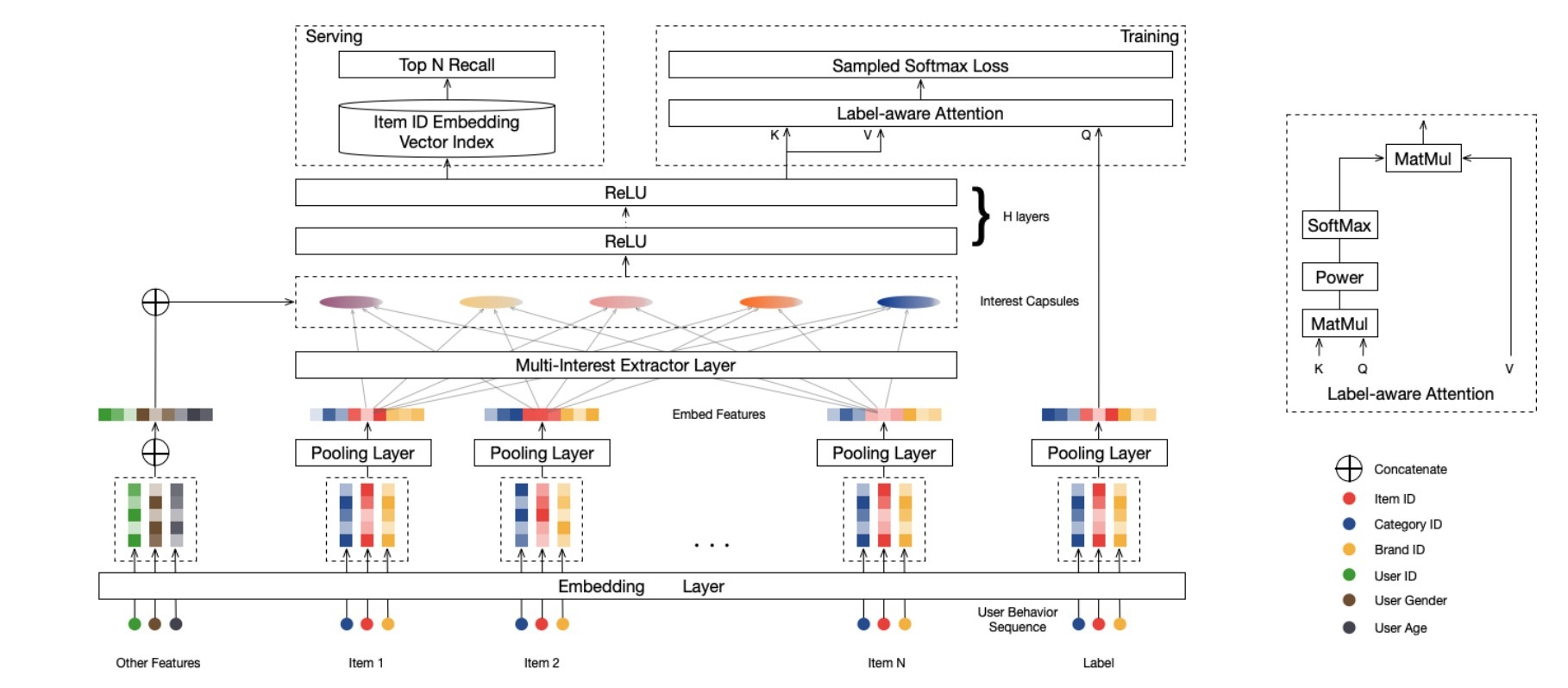## 3.3 Multi-Interest Extractor Layer

### 3.3.1 Dynamic Routing

$\vec{c}_i^l \in R^{N_l \times 1}, i \in \lbrace 1,\cdots,m \rbrace$

high-level capsules $j \in \lbrace 1, \cdots, n \rbrace$，它相应的向量为：

$\vec{c}_j^h \in R^{N_h \times 1}, j \in \lbrace 1, ..., n \rbrace$

$b_{ij} = (\vec{c}_j^h)^T S_{ij} \vec{c}_i^l$

…(4)

$\vec{z}_j^h = \sum\limits_{i=1}^m w_{ij} S_{ij} \vec{c}_i^l$

…(5)

$w_{ij} = \frac{exp (b_{ij})}{ \sum\limits_{k=1}^m exp (b_{ik})}$

…(6)

$\vec{c}_j^h = squash(\vec{z}_j^h) = \frac{\| \vec{z}_j^h \|^2}{ 1 + \| \vec{z}_j^h \|^2} \frac{\vec{z}_j^h}{ \| \vec{z}_j^h \|}$

…(7)

$b_{ij}$的值被初始化为0, routing process通常会重复三次以便收敛。当routing完成时，high-level capsule的值$\vec{c}_j^h$是确定的，可以被当作是next layers的inputs。

### 3.3.2 B2I Dynamic Routing

1.Shared bilinear mapping matrix.

• 一方面，用户行为是变长的，对tmall用户来说，范围从几十到几百不等，因而，使用固定的bilinear mapping matrix是可泛化推广（generalizable）的
• 另一方面，我们希望interest capsules位于相同的向量空间中，但不同的bilinear mapping matrice会将interest capsules映射到不同的向量空间上。从而，routing logit可以通过以下公式计算：
$b_{ij} = \vec{u}_j^T S \vec{e}_i, i \in I_u, j \in \lbrace 1, \cdots, \rbrace$

…(8)

• $\vec{e}_i \in R^d$表示behavior item i的embedding
• $\vec{u}_j \in R^d$表示interest capsule j的向量。
• bilinear mapping matrix $S \in R^{d \times d}$是跨每个(behavior capsules, interest capsules) pairs间共享的。

2.随机初始化routing logits

3.动态兴趣数(Dynamic interest number)

$K_u' = max(1, min(K, log_2(|I_u|)))$

…(9)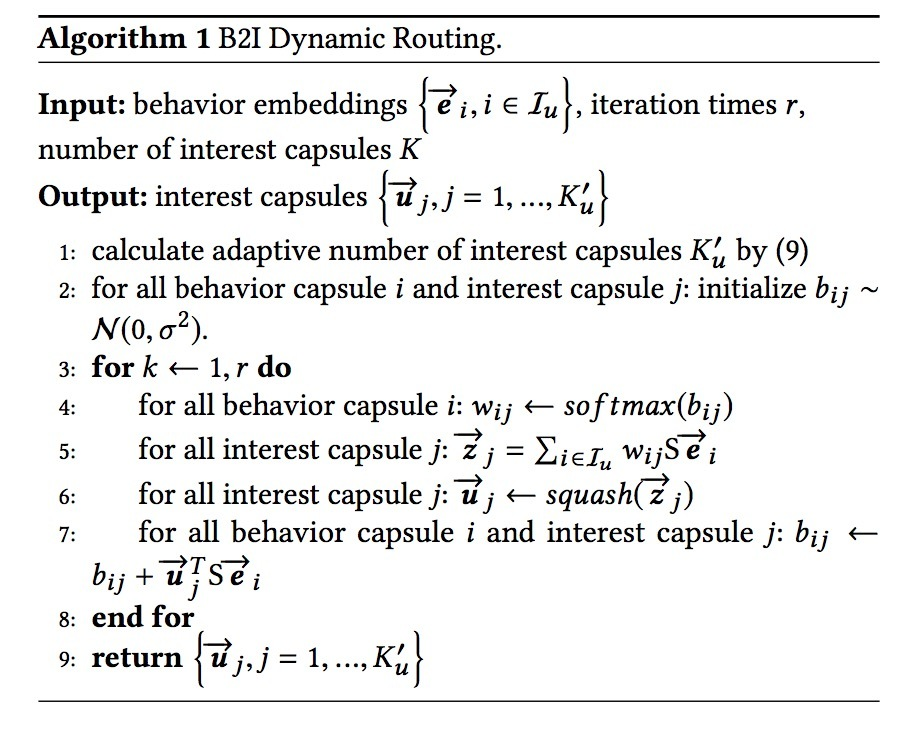## 3.4 Label-aware Attention Layer

\begin{align} \vec{v}_u & = Attention (\vec{e}_i, V_u, V_u) \\ & = V_u softmax(pow(V_u^T \vec{e}_i, p)) \end{align}

• pow表示element-wise指数操作符(exponentiation oprator)，pow(x,y)表示x的y次幂；
• p是一个可调参数，用于调节attention分布。当p接近0时，每个interest capsule趋向于接收偶数(even)attention。当p大于1时，随着p的增加，该值会大于点乘，会接受越来越多的权重。考虑到极限的情况，当p无穷大时，attention机制会变成一种hard attention，它会选中具有最大attention的值，并忽略其它。在我们的实验中，我们发现：使用hard attention会导致更快的收敛。

• $\vec{e}_i$表示target item i的embedding
• $V_u$：用户u的表示向量，共由K个interest capsules组成
• $\vec{v}_u$：user u对于item i的output vector

## 3.5 Training & Serving

$Pr(i | u) = Pr(\vec{e}_i | \vec{v}_u) = \frac{exp(\vec{v}_u^T \vec{e}_i)}{ \sum_{j \in I} exp(\vec{v}_u^T \vec{e}_j)}$

…(10)

$L = \sum\limits_{(u,i) \in D} log Pr(i|u)$

…(11)

## 3.6 与已存在方法的联系

DIN. DIN可以捕获用户的多样化兴趣，MIND和DIN具有相似的目标。然而，这两种方法在达成该目标以及应用上各不相同。为了处理多样化兴趣，DIN会在item级别使用一个attention机制，而MIND使用dynamic routing来生成interest capsules，并在interest level考虑多样性。再者，DIN关注在ranking stage处理上千的items，而MIND会解耦inferring用户表示和衡量user-item兼容性，使它应用于在matching stage上海量items的召回。

# 4.实验

## 4.2 超参数分析

routing logits的初始化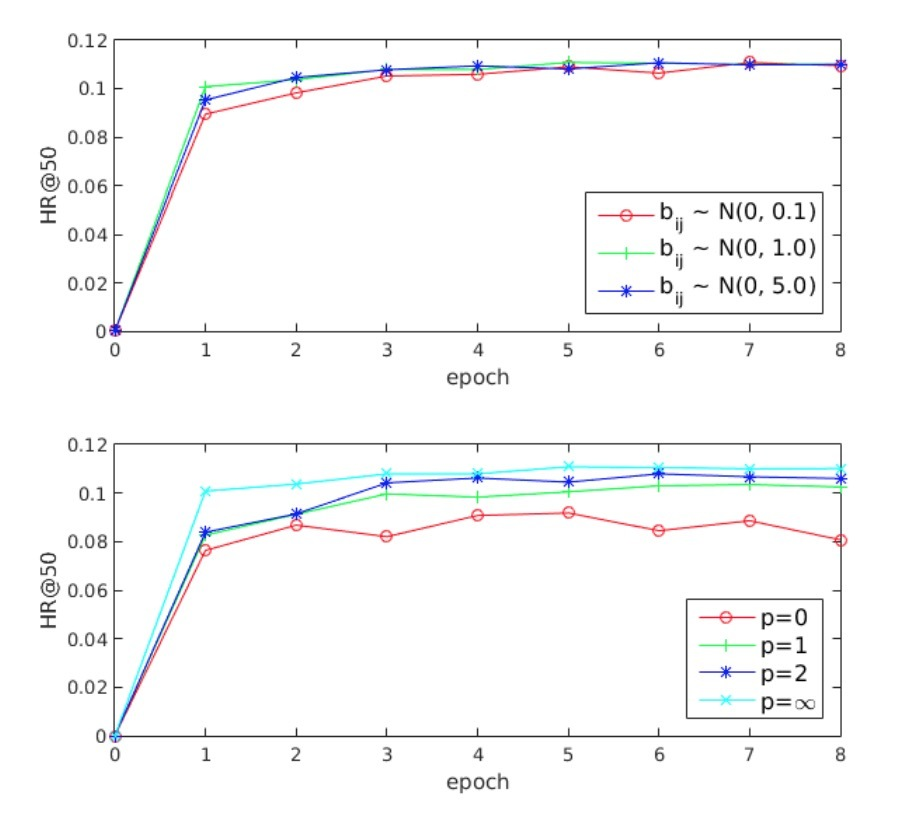## 4.3 在线实验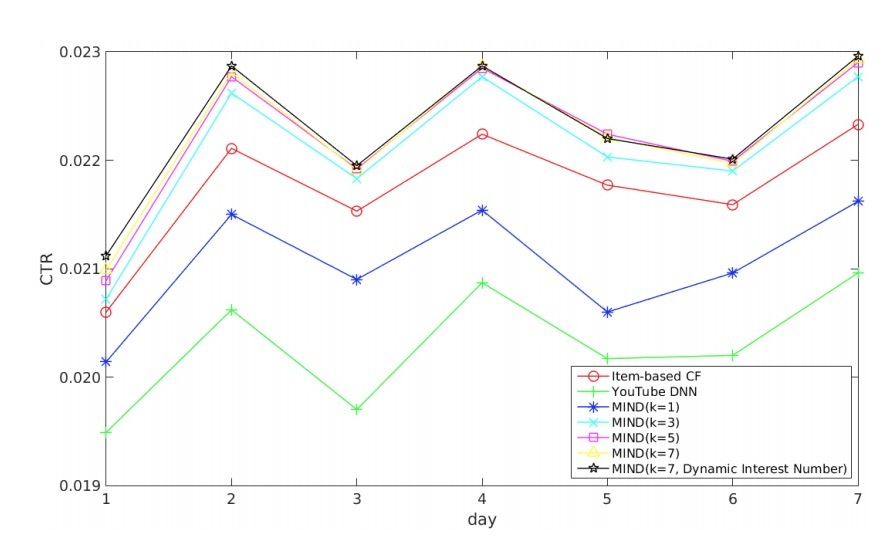• 2) 另一个显著的趋势是，MIND的效果会随着抽取的兴趣数的增加而变好（从1到5）。
• 3) 当抽取的兴趣数为5时，MIND的效果达到峰值，这之后，CTR保持数据，兴趣数达到7的提升可以忽略。
• 4) 使用动态兴趣数(dynamic interest number)的MIND与具有7个兴趣的MIND效果相当。

• 首先，对于Tmall，用户兴趣的最优数目是5-7, 这可以表示用户兴趣的平均多样性（diversity）。
• 第二，动态兴趣数机制并不能带来CTR增益，但在实验期间，我们意识到该scheme可以减少serving的开销，这有利于tmall这样的大规模服务，在实际上更易接受。

## 4.4 案例研究

### 4.4.1 耦合系数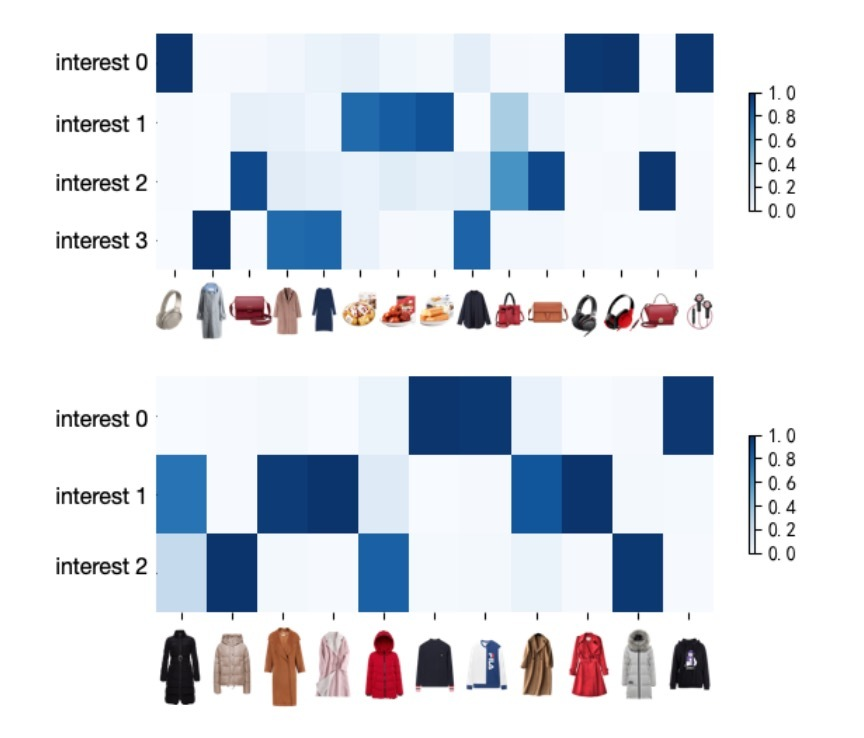### 4.4.2 item分布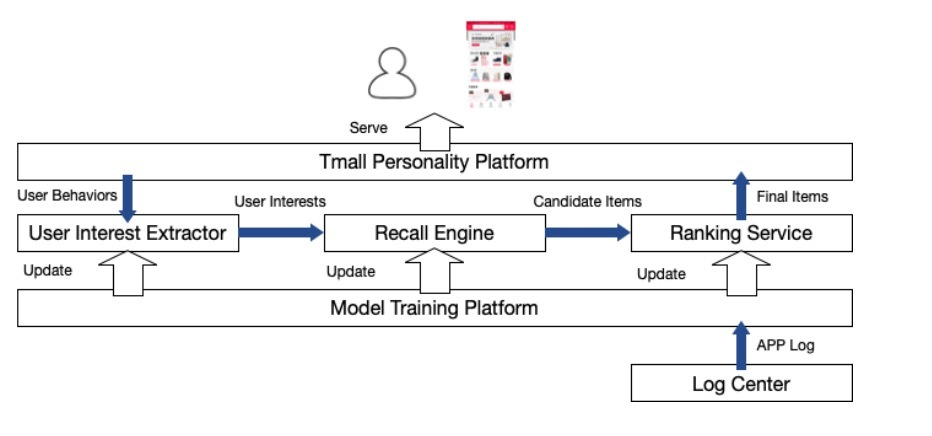# 5.系统部署